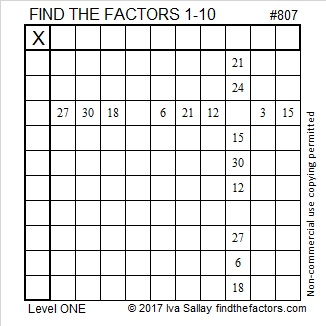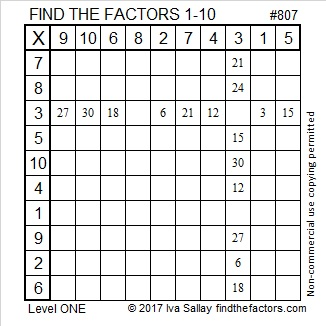# 807 and Level 1

What can I say about the number 807?

807 is palindrome 151 in BASE 26 because 1(26²) + 5(26) + 1(1) = 807.

Anything else? Well, I can figure out a few other things because 807’s has two prime factors, 3 and 269:

We can write ANY number (unless it’s a power of 2) as the sum of consecutive numbers in at least one way. 807 has three different ways to do that:

• 403 + 404 = 807 because 807 isn’t divisible by 2.
• 268 + 269 + 270 = 807 because it is divisible by 3.
• 132 + 133 + 134 + 135 + 136 + 137 = 807 since it is divisible by 3 but not by 6.

I know that one of 807’s factors, 269, is a hypotenuse of a Pythagorean triple, so 807 is also. Thus. . .

• (3·69)² + (3·260)² = (3·269)², or in other words, 207² + 780² = 807²

Since 807 has two odd sets of factor pairs, I know that 807 can be written as the difference of two squares two different ways:

• 136² – 133² = 807
• 404² – 403² = 807

I don’t usually do this, but today’s puzzle has something in common with 807. Can you tell what it is?Print the puzzles or type the solution on this excel file: 10-factors 807-814

• 807 is a composite number.
• Prime factorization: 807 = 3 x 269
• The exponents in the prime factorization are 1 and 1. Adding one to each and multiplying we get (1 + 1)(1 + 1) = 2 x 2 = 4. Therefore 807 has exactly 4 factors.
• Factors of 807: 1, 3, 269, 807
• Factor pairs: 807 = 1 x 807 or 3 x 269
• 807 has no square factors that allow its square root to be simplified. √807 ≈ 28.4077454## One thought on “807 and Level 1”

1.theintrovertman

I really appreciate it.👌👌👌👌👏👏👏🍀🍀

This site uses Akismet to reduce spam. Learn how your comment data is processed.